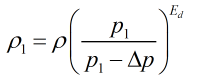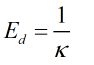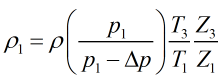# C150 – Upstream Density Calculation

## Description

This calculation corrects the density to upstream conditions.

IP Petroleum Measurement Manuel: Part VII Section 2: Continuous Density Measurement

Implementation of ISO 5167:2003 at Gas Terminals for Sales Gas Metering Systems using Densitometers in the ‘bypass’ mode. Dti March 2007.

Kelton calculation reference C150

FLOCALC calculation reference F037

KIMS calculation reference K151

## Options

### Method

• Density exponent

This option calculates the upstream density using either the density or isentropic exponent as an input.

• Isenthalpic

This option calculates the upstream density using upstream and downstream temperatures and compressibility as inputs.

### Density correction exponent

• Calculate from isentropic exponent

Check the box if you want to enter the isentropic exponent for use in the calculation.

## Calculation

All calculations are performed in SI units unless otherwise stated.

### Upstream density

When density exponent is the selcted method, the upstream density is calculated by:Whereρ = Downstream density p1 = Upstream pressure Δp = Differential pressure Ed = Density exponent κ = Isentropic exponent

When isenthalpic is the selected method, the upstream density is calculated by:Where ρ = Downstream density (measured density at P2 T3 from the densitometer in bypass mode) p1 = Upstream pressure Δp = Differential pressure T1 = Upstream temperature T3 = Measured temperature at the downstream recovery point Z1 = AGA 8 Compressibility at P1,T1 Z3 = AGA 8 Compressibility at P2,T3

Back